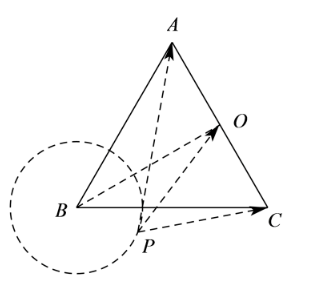$\text{A.}$ $1$ $\text{B.}$ $\sqrt{2}$ $\text{C.}$ $\sqrt{3}$ $\text{D.}$ $2$
【答案】 A

【解析】$$\therefore(\overrightarrow{A P} \cdot \overrightarrow{C P})_{\min }=4-3=1 \text {. }$$